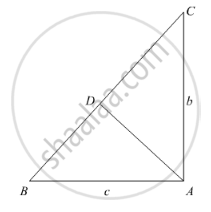Share

Books Shortlist
Your shortlist is empty

# Solution for Using Pythagoras Theorem Determine the Length of Ad in Terms of B and C Shown in Figure. - CBSE Class 10 - Mathematics

ConceptApplication of Pythagoras Theorem in Acute Angle and Obtuse Angle

#### Question

Using Pythagoras theorem determine the length of AD in terms of b and c shown in Figure.

#### SolutionWe have,

In ΔBAC, by Pythagoras theorem

BC2 = AB2 + AC2

⇒ BC2 = c2 + b2

⇒ BC = sqrt(c^2+b^2)             ........(i)

In ΔABD and ΔCBA

∠B = ∠B                             [Common]

∠ADB = ∠BAC                     [Each 90°]

Then, ΔABD ~ ΔCBA           [By AA similarity]

therefore"AB"/"CB"="AD"/"CA"           [Corresponding parts of similar Δ are proportional]

rArrc/sqrt(c^2+b^2)="AD"/b

rArr"AD"="bc"/sqrt(c^2+b^2)

Is there an error in this question or solution?

#### Video TutorialsVIEW ALL 

Solution for question: Using Pythagoras Theorem Determine the Length of Ad in Terms of B and C Shown in Figure. concept: Application of Pythagoras Theorem in Acute Angle and Obtuse Angle. For the course CBSE
S